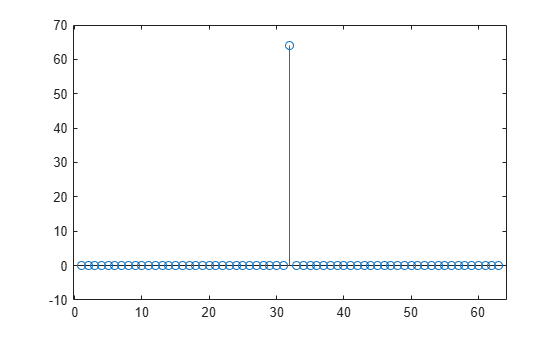# wlanGolaySequence

Generate Golay sequence

## Syntax

``[Ga,Gb] = wlanGolaySequence(len) ``

## Description

example

````[Ga,Gb] = wlanGolaySequence(len) `returns the Golay sequences `Ga` and `Gb` for a specified sequence length. The sequences are defined in IEEE® 802.11ad™-2012, Section 21.11.```

## Examples

collapse all

Generate complementary 32-length Golay sequences.

`[Ga,Gb] = wlanGolaySequence(32);`

The sum of the autocorrelations is a dirac delta function.

```figure stem(xcorr(Ga)+xcorr(Gb))```## Input Arguments

collapse all

Sequence length, specified as 32, 64, or 128.

Data Types: `double`

## Output Arguments

collapse all

Golay sequence, returned as a column vector of integers of length `len`.

Complementary Golay sequence, returned as a column vector of integers of length `len`.

## Version History

Introduced in R2017b